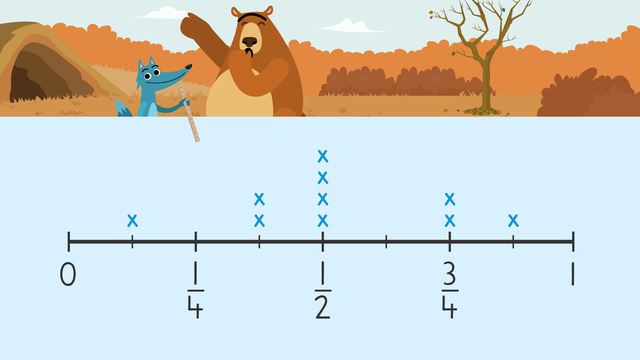# Fractions on Line PlotsRating

Ø 5.0 / 6 ratings
The authorsTeam Digital
Fractions on Line Plots
CCSS.MATH.CONTENT.4.MD.B.4

## Line Plots with Fractions

Line plots with fractions are a type of graph that shows data on a fractional number line. There are x’s created above the line to indicate the frequency of each unit of data.

## Line Plots with Fractions – Example

In this example, we want to look at how much snow fell on different days. We have given data and the question is “What was the least amount of snow accumulated in a day?” With the help of using line plots, we can answer questions about the data.Our line does not indicate the fraction for the day with the least amount of snow, so we need to count how many increments the line is divided into. The line plot is divided into eighths, so the denominator of our fraction will be 8. Our date is on the first increment on the line plot. The position of the increment is the numerator of the fraction we want to find out. Combining our data, we see that numerator 1 and denominator 8 are the fraction $\frac{1}{8}$, so the least amount of snow fallen in a day is $\frac{1}{8}$ feet!

If the question now commands us to find the greatest amount of snowfall accumulated in a day, we look at our line plot again and search for the rightmost entry on the line.Using our counted increments from earlier, we see that the greatest amount of snowfall in a day accumulated up to $\frac{7}{8}$ feet of snow!

## Line Plots with Fractions – Summary of Steps

Step # What to do
1 Look at the data on the line plot to understand
what you need to find.
2 If the line does not indicate the fraction you
are looking for, count the number of increments
on the line plot.
3 The number of increments total is the denominator
and the specific increment is the numerator

## Line Plots with Fractions – Further Practice

Now it’s your turn! What is the difference in the amounts of snow between the greatest and the least?There will be additional activities with line plots with fractions worksheets and exercises. These line plots with fractions 4th-grade worksheets will provide you with continued practice using line plots to record data and answer questions about the fractional data.

### TranscriptFractions on Line Plots

1.good

From Hailey Lopez, 6 months ago
2.so cool but its sofatutor so its cool

From Averie, 7 months ago
3.COOL.

From Ninja Yousif, 8 months ago

## Fractions on Line Plots exercise

Would you like to apply the knowledge you’ve learned? You can review and practice it with the tasks for the video Fractions on Line Plots.
• ### Nari needs your help.

Hints

What is the first step for answering questions about fractions on a line plot?

What is the last step for answering questions about fractions on a line plot?

Solution
• Plot data on the line plot.
• Read questions and identify keywords that tell you what to look for on the line plot.
• Count how many increments the line is divided into if the fraction is not listed on the line plot.
• Simplify fractions as necessary.
• ### There is a lot of snow!

Hints

What does greatest amount of snow accumulation mean?

Which fraction on the line plot represents the most snowfall accumulation?

Make sure to simplify the fraction.

Solution
• The greatest amount of snowfall accumulated in a day was $\frac{8}{8}$.
• $\frac{8}{8}$ is simplified to 1.
• The answer is 1 foot.
• ### Find the difference.

Hints

What does difference mean?

Solution
• Difference means to subtract so you need to find the fractions on the line plot that represent the least and greatest amount of snowfall accumulation and subtract them.
• $\frac{1}{8}$ is the least.
• $\frac{8}{8}$ is the greatest.
• $\frac{8}{8}$ - $\frac{1}{8}$ is $\frac{7}{8}$.
• The answer is $\frac{7}{8}$.
• ### Nari needs to combine fractions.

Hints

Simplify the fractions on the line plot to figure out which fractions are less than $\frac{1}{4}$.

What does combine mean?

Solution
• Combine means to add so you need to add the fractions that represent less than $\frac{1}{4}$ of a foot of snow on the line plot.
• $\frac{1}{8}$ is less than $\frac{1}{4}$ of a foot of snow.
• You add $\frac{1}{8}$ two times since there are two Xs above $\frac{1}{8}$ on the line plot.
• $\frac{1}{8}$ + $\frac{1}{8}$ = $\frac{2}{8}$
• $\frac{2}{8}$ is simplified by dividing the numbers by 2.
• The answer is $\frac{1}{4}$.
• ### Nari plots snowfall accumulation.

Hints

Which data point on the line plot illustrates the least amount of snowfall accumulation?

Which data point on the line plot illustrates the most amount of snowfall accumulation?

Solution

The image pictured illustrates the fractions in order from least to greatest on the line plot.

• ### Nari has a tough problem to solve.

Hints

What does combine tell you to do?

What does the difference tell you to do?

• $\frac{1}{8}$ + $\frac{1}{8}$ = $\frac{2}{8}$
• $\frac{8}{8}$ - $\frac{2}{8}$ = $\frac{6}{8}$
• Finally, you need to simplify $\frac{6}{8}$ since 6 and 8 can be divided by 2.
• The answer is $\frac{3}{4}$.